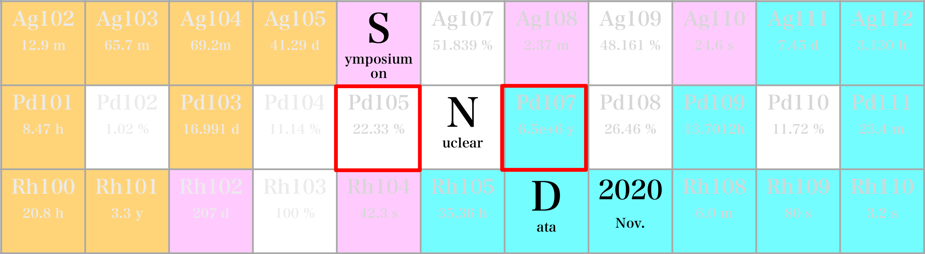#Symposium on Nuclear Data 2020

26-27 November 2020
RIKEN Wako Campus
Asia/Tokyo timezone

## Introduction to Nuclear Reactor Theory

Not scheduled
30m
RIBF201 (RIKEN Wako Campus)

### RIBF201

#### RIKEN Wako Campus

Hirosawa 2-1, Wako City, Saitama 351-0198, Japan
Oral Presentation

### Speaker

Tomohiro Endo (Nagoya University)

### Description

In this tutorial, I would like to briefly introduce the nuclear reactor theory.
Please keep in mind that the whole nuclear fission power plant is a too complicated system, thus it is not easy to understand the multi-physics in the nuclear power plant. From the overall view of the nuclear power plant, thermal energy via fission in the nuclear reactor core is utilized to finally generate electricity.

To appropriately design and control the nuclear reactor, we must understand and predict the behavior of neutrons and nuclear reactions. For this purpose, reactor physics has been developing as an engineering science to systematize the relevant knowledge.
The effective neutron multiplication factor $k_{\mathrm{eff}}$ is an important core-characteristics parameter to judge whether the nuclear reactor core is subcritical, critical, or supercritical. The $k_{\mathrm{eff}}$ value indicates the ratio of fission-induced neutron-production to neutron loss (absorption or leakage). In the nuclear reactor, fission power is constantly maintained at the “critical state” of the fission chain reaction.
To roughly predict the neutron multiplicity by the fission chain reaction, the point kinetics equation is simple and useful. If we think of a point kinetic equation without delayed neutrons, this equation is similar to that of the Susceptible-Infected-Removed (SIR) model, which is used in the epidemiology. However, if there were no delayed neutrons in the fission event, we could never control the fission chain power reaction. In other words, the delayed neutron plays an important role to control the fission power in the actual nuclear reactor core by humans.

In order to predict the macroscopic and average behavior of neutrons, the Boltzmann neutron transport equation is utilized as the master equation. Note that the rigorous Boltzmann equation is too complicated to obtain the solution. At the dawn of the nuclear age, scientists and engineers simplified the prediction model based on ingenious approximations such as diffusion and Fermi-age theories. Recently, as computing performance and computational science improve, we can accurately solve the numerical solution of the Boltzmann equations as much as possible. At the end of this tutorial, I will briefly explain unique numerical analysis methods, which have been developed and utilized in the field of reactor physics. Through this tutorial, I would like to promote understanding that the nuclear data such as microscopic nuclear cross section are essential input data for the reactor physics analysis.

### Primary author

Tomohiro Endo (Nagoya University)

### Presentation Materials

There are no materials yet.